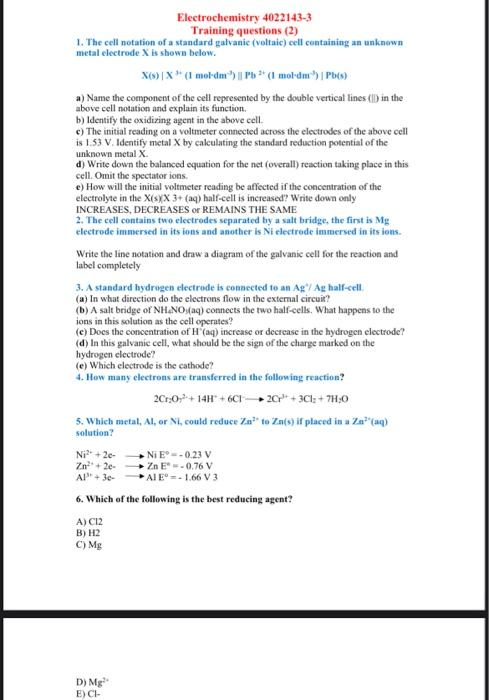Home / Expert Answers / Chemistry / help-electrochemistry-4022143-3-training-questions-2-1-the-cell-notation-of-a-standard-galva-pa751

# (Solved): help Electrochemistry 4022143-3 Training questions (2) 1. The cell notation of a standard galva ...

help ????Electrochemistry 4022143-3 Training questions (2) 1. The cell notation of a standard galvanic (voltaic) eell containing an unknown metal electrode is show below. a) Name the component of the cell represented by the double vertical lines (I) in the above cell notation and explain its function. b) Identify the oxidizing agent in the above cell. c) The initial reading on a voltmeter connected across the electrodes of the above cell is 1.53 . Identify metal by calculating the standard reduction potential of the unknown metal . d) Write down the balanced equation for the net (overall) reaction taking place in this cell. Omit the spectator ions. e) How will the initial voltmeter reading be affected if the concentration of the electrolyte in the half-cell is increased?? Write down only INCREASES, DECREASES or REMAINS THE SAME 2. The cell contains two electrodes separated by a salt bridge, the first is electrode immersed in its ions and another is Ni electrede immersed in its ions. Write the line notation and draw a diagram of the galvanic cell for the reaction and label completely 3. A standard hydrogen electrode is conacted to an half-cell. (a) In what direction do the electrons flow in the extemal circuir? (b) A salt bridge of connects the two half-eells. What happens to the ions in this solution as the cell operates? (c) Does the concentration of (aq) increase or decrease in the hydrogen electrode? (d) In this galvanic cell, what should be the sign of the charge marked on the hydrogen electrode? (e) Which electrode is the cathode? 4. How many electrons are transferred in the following reaction? 5. Which metal, , or , could reduce to if placed in a solution? 6. Which of the following is the best reducing agent? A) B) C)

We have an Answer from Expert